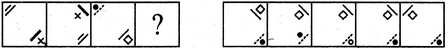# Non Verbal Reasoning - Analogy - Discussion

### Discussion :: Analogy - Section 1 (Q.No.6)

Each of the following questions consists of two sets of figures. Figures A, B, C and D constitute the Problem Set while figures 1, 2, 3, 4 and 5 constitute the Answer Set. There is a definite relationship between figures A and B. Establish a similar relationship between figures C and D by selecting a suitable figure from the Answer Set that would replace the question mark (?) in fig. (D).

6.

Select a suitable figure from the Answer Figures that would replace the question mark (?).(A)     (B)      (C)     (D)                  (1)      (2)      (3)      (4)      (5)

 [A]. 1 [B]. 2 [C]. 3 [D]. 4 [E]. 5

Explanation:

The combination of two symbols placed at the lower-right corner, rotates 90oCW and moves to the Upper-right corner. Also, the combination of two symbols placed at the upper-left corner, moves to the lower-right corner.

 Pallabi said: (Jan 28, 2019) Symbols placed at lower right corner is rotating anti clock wise and moves to upper right corner.

 Ronnie said: (Feb 2, 2019) Yes, you are right @Pallabi.